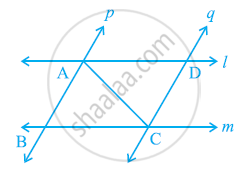# l and m are two parallel lines intersected by another pair of parallel lines p and q (see the given figure). Show that ΔABC ≅ ΔCDA. - Mathematics

l and m are two parallel lines intersected by another pair of parallel lines p and q (see the given figure). Show that ΔABC ≅ ΔCDA.#### Solution

In ΔABC and ΔCDA,

∠BAC = ∠DCA (Alternate interior angles, as p || q)

AC = CA (Common)

∠BCA = ∠DAC (Alternate interior angles, as l || m)

∴ ΔABC ≅ ΔCDA (By ASA congruence rule)

Concept: Criteria for Congruence of Triangles
Is there an error in this question or solution?

#### APPEARS IN

NCERT Class 9 Maths
Chapter 7 Triangles
Exercise 7.1 | Q 4 | Page 119